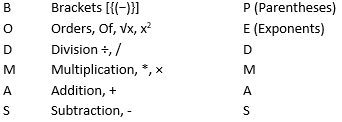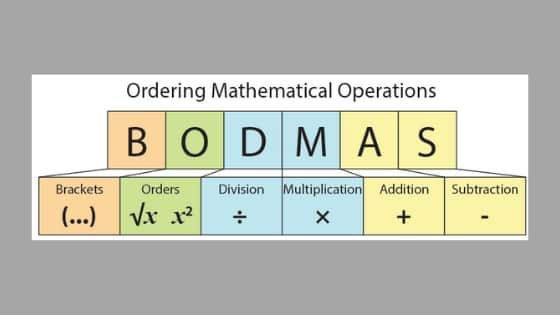Bodmas Rule

What is Bodmas Rule - Order of Operations with Examples

Order of Operations

In a logic-oriented subjects like mathematics, the order of operations is a set of standard rules that reflect conventions about which process to perform first to evaluate a given numerical expression. With a basic summation that just has two numbers and one single operation, or sign, it's easy to calculate the result. Either you perform addition, subtraction, multiplication, or division.

But what if there are several numbers and different operations in an expression at the same time? Maybe you need to multiply and divide or divide and add. What do you do then?

For example, multiplication is given a higher precedence than addition, and it has been this way right from the time when modern algebraic notations were introduced. Thus, the expression 3 + 5 × 2 is interpreted to have the answer 3 + (5 × 2) = 13, not (3 + 5) × 2 = 16. When exponents were introduced in the 16th and 17th centuries, they were given precedence over both addition and multiplication and are used as a superscript to the right of their base. Thus, 2 + 52 = 27 and 2 × 52 = 50.

These conventions exist to eliminate any ambiguity while enabling notation to be as brief as possible. Where it is desired to override the precedence conventions, or just to emphasize them, parentheses (), [ ], { } can indicate an alternate order or reinforce the default order to avoid confusion. For example, (2 + 3) × 4 = 20 emphasize addition to precede multiplication, and (3 + 5)2 = 64 emphasize addition to precede exponentiation.

What is BODMAS Rule?

BODMAS is a short form for Brackets, Of, Division, Multiplication, Addition and Subtraction. In some regions, it is also known as PEDMAS- Parentheses, Exponents, Division, Multiplication, Addition and Subtraction.

This rule explains the order of operations (order of precedence) to solve an expression. According to BODMAS rule, in a given mathematical expression that contains combination of signs: brackets ((), {}, [], −), multiplication, of, addition, subtraction, division, we must first solve or simplify the brackets followed by of (powers and roots etc.), of, then division, multiplication, addition and subtraction from left to right. Solving the problem without following BODMAS rule or from right hand direction will give you a wrong answer.Use of Bracket

Let’s understand the use of brackets first. It is a vital part of forming and evaluating mathematical expressions. For example, let’s say you went to the store and bought 10 oranges. 2 oranges cost Rs. 10 each, 4 of them were Rs 5 each and remaining 4 were for Rs. 20 each. Now how to represent this mathematically?

The equation will be something like this:
2 × 10 + 5 × 4 + 4 × 20 = 120

But the above equation appears very confusing, doesn’t it? While evaluating this expression, you will be confused which operation to do first and you might make errors. So how to represent this expression in a better way using brackets?

(2 × 10) + (5 × 4) + (4 × 20) = 120

This makes much more sense, right? It is an organized representation of the expression. Now you will have a better understanding of the order of operation. The use of brackets is a must in mathematics.

BODMAS rule

Let’s understand BODMAS rule with the help mathematical expressions. In the above situation, we performed two operations which are addition and multiplication. Suppose in an equation you have to perform more such operations; then how will you decide which operation to perform first? For example, the following equation:

25 × 5 + 46 ÷ 2 = ?

In this expression, BODMAS rule comes handy. It gives us an understanding of the order of operation or precedence. Here, according to BODMAS rule, first we need to perform division, followed by multiplication, and then addition.

46 ÷ 2 = 23
25 × 5 = 125
125 + 23 = 148

Solved examples

Question: 5 × (4 + 3) =?
Options:
(a) 65
(b) 25
(c) 35
(d) 45

Solution:

According to BODMAS rule,
5 × (4 + 3)
= 5 × (7)
= 35

Question: Solve for y using BODMAS rule.
36 ÷ 2 + y × 3 − 22 = 8
Options:
(a) 2
(b) 8
(c) 4
(d) 1

Solution:

36 ÷ 2 + y × 3 −22 = 8
⇒ 18 + 3y – 22 = 8
⇒ 18 + 3y = 30
⇒ 3y = 30 − 18
⇒ 3y = 12
⇒ y = 12/3
⇒ y = 4

Special Cases

Serial Exponentiation:

An ambiguity occurs in the case of serial exponentiation, for example, the expression ${4^{{3^2}}}$. The expression ${4^{{3^2}}}$ should not be evaluated as 4096. The correct solution is ${4^{\left( {{3^2}} \right)}} = 262144$.

Follow the top-down order of execution:
${a^{{b^c}}} = {a^{\left( {{b^c}} \right)}}$
Note: This should not be confused with the following case.
${\left( {{a^b}} \right)^c} = {a^{bc}}$

Example: Find the value of ${2^{{3^2}}}$.

Solution:
${2^{{3^2}}} = {2^9} = 512$

Serial Division:

A similar kind of ambiguity is observed in the case of serial division, for example, consider the expression 200 ÷ 10 ÷ 2 which can be misinterpreted as 200 ÷ 10 ÷ 2 = 40

But if you follow the rule of BODMAS where one should move from left to right, the correct answer will be found which in this case is:
200 ÷ 10 ÷ 2 = 10

Some examples:
Question: Simplify 4 + 4 of 4 ÷ 4 of 4 × 4.
Options:
(a) 47
(b) 68
(c) 1
(d) 4

Solution:

Applying the BODMAS rule,
4 + 4 of 4 ÷ 4 of 4 × 4
= 4 + (4 of 4) ÷ 4 of 4 × 4
= 4 + 16 ÷ 4 of 4 × 4
= 4 + 4 of 4 × 4
= 4 + (4 of 4) × 4
= 4 + 16 × 4 = 4 + 64
= 68

Question: In the given equation find which of the following interchange of signs/numbers will make the equation 8 + 7 × 1 = 7 - 2 correct.
Options:
(a) + with –
(b) + with ×
(c) × with –
(d) None of these

Solution:

If we substitute ‘×’ in the place of ‘− ‘and ‘− ‘ in the place of ‘×’ then the both the sides of the equation get balanced.
On the left hand side,
8 + 7 × 1
Substitute ‘− ‘ in the place of ‘×’
8 + 7 – 1 = 15 − 1 = 14

On the right hand side,
7 - 2
Substitute ‘×’ in the place of ‘− ‘
7 × 2 = 14
Therefore, LHS=RHS.

Question: Find the value of ${3^{{3^2}}}$
Options:
(a) 19683
(b) 29683
(c) 19863
(d) 29863

Solution:

${3^{{3^2}}} = {3^9} = 19683$

Question: Find (1/4 + 7/4) – 2 = ?
Options:
(a) 1
(b) 2
(c) 0
(d) 4

Solution:
(1/4 + 7/4) - 2
= (8/4) - 2
= 2-2
= 0

Practice Sheet:
Evaluate (Level 1)
6 × 9 + 8
122 – 18 ÷ 2
(16 + 15) × 2
8 + 9 x (1 + 7) ÷ 8 - 6
18 - 2(20 + 16 ÷ 4 x 3 - 2 x 2) + 10
6 + [(18 - 9) ÷ (3² + 3)] – 3
(84 ÷ 12) + 21 x (12 + 8) ÷ 2
(63 + 15) ÷ (3 x 4) - 24 + 8
77 ÷ 11 + (18 - 6) x 9
(17 + 18) x 3 ÷ (2 + 13) – 28

Find the missing operator * (Level 2)
(18 - 6) * 2 = 6
3 x 5 * 2 = 17
(10 - 6) * 2 = 8
6 * (9 ÷ 3) = 18
20 + 5 * 5 = 45
(13 - 5) * 2 = 4
9 * (8 ÷ 4) = 11
9 * (6 ÷ 2) = 27
3 x 8 * 2 = 12
83 + 2 * 8 = 99
(8 + 2) * 5 = 2
(20 - 5) * 3 = 5

Evaluate (Level 3)
2[2 + 2{39 - 2(17 + 2)}]
3 x 7 + 4 – 6 ÷ 3 – 7 + 45 ÷ 5 x 4 + 49
(202 + 60 × 10) – 5000
3{35 + (45 + 10 ÷ 2 × 3 - 50) + 5}
75 % of 480 + 20 % of 540 - 100
25(-4) + 500 ÷ (70 + 5 × 6)

Solve for p using BODMAS rule (Level 4)
2p - 4 = 10
5p - 6 = 3p - 8
2(3p - 7) + 4(3p + 2) = 6 (5p + 9) + 3
p + 9 = 18 - 2p
4p + 6 = -10
2p + 6 = 4p - 2
-p - 1 = 221 + 2p
15 + 5p = 0
17p - 12 = 114 + 3p
2p - 10 = 10 - 3p
12p + 0 = 144

Simplify (Level 5)
60 = ? % of 65
0.81 of ? = 40.5
0.29 of ? = 26.1
2450 ÷ 7 + 112 × 2.5 = ? × 2
125 % of 7/6 of ? = 84 % of 1250
4/7 of 441 ÷ 18 + 14 = √?
6/5 of 7/8 × 24/21 of 2425 = ?
(243)2 ÷ (27)2 × 32 ÷ 12 = ?
58.33 % of 408 + 62.5 % of 360 = 18 × ?
544 ÷ 16 × 32 – 766 + 224 = ?
333.33 + 33.33 + 13 + 0.33 + 0.03 = ?
525 – (13)² + 63 = (?)² + 95
(52.5 x 18 x 4) ÷ 9 + 92 = (?)3
28 × 2 × 864 ÷ √2916 = ? + 540
√3100 × √5657 ÷ √250 = ? ÷ 8
89.988 % of 699.9 + 50.002 % of 999.99 – 170.015 =?
6999 ÷ 70.005 × 94.998 = ? × 19.999
7441 ÷ 34 × 12 = ? × 9 + 110
4200 – 35 × 2242 ÷ 59 = ? × 14
1224 × ? = (408)2
√ (√42849 + √54756) = ?
88 % of 1850 + 35 % of 2400 = ? + 758
76 % of 960 – 45 % of 148 =? % of 5525
(4096)(3.7) ÷ (256)(4.3) × (64)5 ÷ (16) – 4 = (4)?
(32128 + 18372 + 2180) ÷ 30 = ?
6.8 × 8.8 × 11.9 – 202.596 = ?
3111.3 × 314.7 = 312 × ?
(1024 – 362 – 214) ÷ (786 – 730) = ?
40.5 × 7 × 4 ÷ 6 – (8)2 = (?)3
69.39 – 58.46 + 79.98 = ? + 76.92
2.9 × 8.41 + 9.261 + 18.27 × 5.0 = ?
56 % of 616 – ? % of 482 = 103.96
5566 – 7788 + 9988 = ? + 4444
56 × 15 ÷ 3 – 83 = ? – 139
(6) 4 ÷ (36) 3 × 216 = 6(? – 5)
√1444 + √5184 = 22 × ?